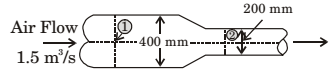## Fluid Mechanics and Hydraulic Machinery Miscellaneous

#### Fluid Mechanics and Hydraulic Machinery

1. Air flows at the rate of 1.5 m3/s through a horizontal pipe with a gradually reducing crosssection as shown in the figure. The two crosssections of the pipe have diameters of 400 mm and 200 mm. Take the air density as 1.2 kg/m3 and assume inviscid incompressible flow. The change in pressure (p2 – p1) (in kPa) between sections 1 and 2 is1.  P1 + V1² + Z1 = P2 + V2² + Z2 [Z = constant] δg 2g δg 2g

 A1 = π × 0.4² = 0.1256 m² 2

 A2 = π × 0.2² = 0.314 m² 4

 P2 − P1 = V1² − V2² δg 2g

 P2 − P1 = 1Q2 − Q21.2 2 A1² A2²

 P2 − P1 = 0.6 × (1.5)²1 − 1A1² A2²

 P2 − P1 = 1.351 − 10.01577 0.00985

= 1.35 [63.41 – 1015.22]
= –1.28 ×10³ Pascal
= – 1.28 kPa

##### Correct Option: A

 P1 + V1² + Z1 = P2 + V2² + Z2 [Z = constant] δg 2g δg 2g

 A1 = π × 0.4² = 0.1256 m² 2

 A2 = π × 0.2² = 0.314 m² 4

 P2 − P1 = V1² − V2² δg 2g

 P2 − P1 = 1Q2 − Q21.2 2 A1² A2²

 P2 − P1 = 0.6 × (1.5)²1 − 1A1² A2²

 P2 − P1 = 1.351 − 10.01577 0.00985

= 1.35 [63.41 – 1015.22]
= –1.28 ×10³ Pascal
= – 1.28 kPa

1. Within a boundary layer for a steady incompressible flow, the Bernoulli equation

1. Bernoulli equation does not hold because the flow is frictional.

##### Correct Option: D

Bernoulli equation does not hold because the flow is frictional.

1. For a fluid element in a two dimensional flow field (x-y plane), if it will undergo

1. NA

##### Correct Option: C

NA

1. Streamlines, path lines and streak lines are virtually identical for

1. NA

##### Correct Option: C

NA

1. A velocity field is given as
VV 3x²yî − 6xyzk̂
where x, y, z are in m and V in m/s. Determine if
(i) It represents an incompressible flow
(ii) The flow is irrotational

1. V = 3x²î − 6xyzk̂

 ∂u + ∂v + ∂w = 6xy + 0 − 6xy = 0 ∂x ∂y ∂z

Flow is incompressible
 wy = 1∂u − ∂w2 ∂z ∂x

 = 1 [0 − (−6yz)] 2

wy = 3yz
Flow is rotational. Flow field is independent of time since terms does not include time ‘t’.

##### Correct Option: A

V = 3x²î − 6xyzk̂

 ∂u + ∂v + ∂w = 6xy + 0 − 6xy = 0 ∂x ∂y ∂z

Flow is incompressible
 wy = 1∂u − ∂w2 ∂z ∂x

 = 1 [0 − (−6yz)] 2

wy = 3yz
Flow is rotational. Flow field is independent of time since terms does not include time ‘t’.